# JP4593430B2 - Receiving machine - Google Patents

## Info

Publication number
JP4593430B2
JP4593430B2 JP2005294444A JP2005294444A JP4593430B2 JP 4593430 B2 JP4593430 B2 JP 4593430B2 JP 2005294444 A JP2005294444 A JP 2005294444A JP 2005294444 A JP2005294444 A JP 2005294444A JP 4593430 B2 JP4593430 B2 JP 4593430B2
Authority
JP
Japan
Prior art keywords
signal
frequency
calibration
mismatch
component
Prior art date
Legal status (The legal status is an assumption and is not a legal conclusion. Google has not performed a legal analysis and makes no representation as to the accuracy of the status listed.)
Expired - Fee Related
Application number
JP2005294444A
Other languages
Japanese (ja)
Other versions
JP2007104522A (en
Inventor

Original Assignee
ルネサスエレクトロニクス株式会社
Priority date (The priority date is an assumption and is not a legal conclusion. Google has not performed a legal analysis and makes no representation as to the accuracy of the date listed.)
Filing date
Publication date
Application filed by ルネサスエレクトロニクス株式会社 filed Critical ルネサスエレクトロニクス株式会社
Priority to JP2005294444A priority Critical patent/JP4593430B2/en
Publication of JP2007104522A publication Critical patent/JP2007104522A/en
Application granted granted Critical
Publication of JP4593430B2 publication Critical patent/JP4593430B2/en
Application status is Expired - Fee Related legal-status Critical
Anticipated expiration legal-status Critical

## Images

•••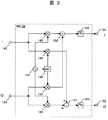•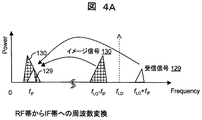•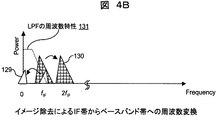•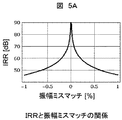•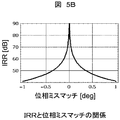•••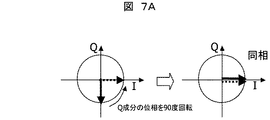•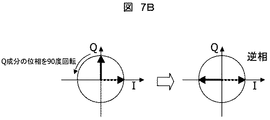•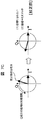•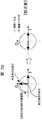•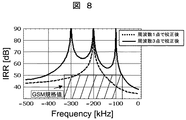••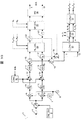•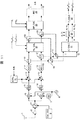•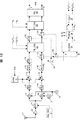•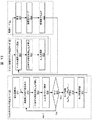••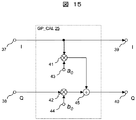•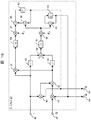•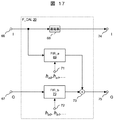••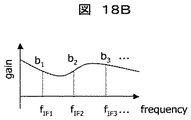••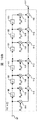•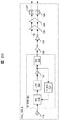••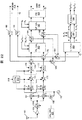•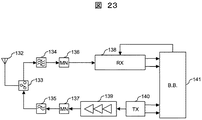•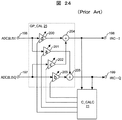•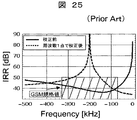## Classifications

• HELECTRICITY
• H04ELECTRIC COMMUNICATION TECHNIQUE
• H04BTRANSMISSION
• H04B1/00Details of transmission systems, not covered by a single one of groups H04B3/00 - H04B13/00; Details of transmission systems not characterised by the medium used for transmission
• H04B1/16Circuits
• H04B1/30Circuits for homodyne or synchrodyne receivers
• HELECTRICITY
• H03BASIC ELECTRONIC CIRCUITRY
• H03DDEMODULATION OR TRANSFERENCE OF MODULATION FROM ONE CARRIER TO ANOTHER
• H03D3/00Demodulation of angle-, frequency- or phase- modulated oscillations
• H03D3/007Demodulation of angle-, frequency- or phase- modulated oscillations by converting the oscillations into two quadrature related signals
• H03D3/009Compensating quadrature phase or amplitude imbalances

## Description

The present invention relates to a receiver and a portable wireless terminal in a wireless system, and more particularly to a technique for calibrating an amplitude mismatch and a phase mismatch between an in-phase (I) component and a quadrature (Q) component in a quadrature bitone signal at a plurality of frequencies.

Patent Document 1 is a document describing a technique for detecting the phase and amplitude mismatch of an orthogonal bitone signal and calibrating the mismatch using the detection result in order to perform high-accuracy image removal in a low-IF receiver. is there. In particular, FIG. 6B of Patent Document 1 shows an example of an IQ mismatch correction circuit.

US2005 / 0070236 A1

Data to be transmitted is divided into an in-phase (I) component and a quadrature (Q) component, and then a carrier wave having a phase difference of 90 degrees is modulated on each of these to modulate a radio frequency (hereinafter abbreviated as “RF (Radio Frequency)”) A wireless system that generates a quadrature modulation signal is adopted in a cellular phone or the like.

In such a wireless system, in recent years, instead of the conventional superheterodyne system, an RF reception signal is set to a low frequency near DC, for example, an intermediate frequency (hereinafter abbreviated as “IF (Intermediate Frequency)”) of 100 kHz to 200 kHz. A low-IF reception method for obtaining a baseband signal after conversion has been widely used. This method can reduce the number of external parts compared to the superheterodyne method, and can manufacture a radio device at low cost.

In the low IF method, when a local oscillator that generates a local signal having a frequency f LO (hereinafter, “LO (Local Oscillator)”) is used to frequency-convert an RF reception signal having a frequency of f LO + f IF to IF f IF , frequency relative to the LO frequency in the relation of the received signal and the symmetry f LO -f IF of interference wave (hereinafter, an image signal) is also frequency-converted to a frequency f IF Similarly, a problem that superimposed on the received signals. In such a case, a general filter cannot attenuate only the image signal without degrading the received signal.

Therefore, when an image removal circuit is used when frequency conversion of the received signal from the IF band to the baseband band, the frequency of the image signal is 2f IF, and the image signal superimposed on the received signal can be separated.

However, if there is an amplitude and phase mismatch between the I and Q components in the input signal of the image removal circuit, the accuracy of image removal decreases.

Here, the accuracy of image removal is evaluated by an image rejection ratio (IRR). The IRR is expressed by the power ratio between the received signal and the image signal at the output of the image removing circuit when the received signal and the image signal are input to the image removing circuit with the same power as in the following equation (1). It can be expressed using the amplitude and phase mismatch of the components.

However, the input power to the image rejection circuit, and the same for the received signal and the image signal, the amplitude mismatch and A m, a theta m and phase mismatch.

In the current semiconductor process, it is very difficult to manufacture an inexpensive integrated circuit (IC) while suppressing a mismatch in the accuracy with which an IRR of, for example, 50 dB or more can be obtained. Therefore, in order to apply the low IF reception method in a wireless system having a large image signal, a circuit for calibrating the mismatch between the I component and the Q component in the orthogonal bitone signal is required.

Patent Document 1 described above discloses a method of inputting a calibration tone signal to a receiver, detecting an IQ mismatch in a quadrature bitone signal in a digital circuit, and calculating a correction coefficient for the mismatch using a feedback circuit. Yes. An example of an IQ mismatch correction circuit is shown in FIG. The calibration circuit in FIG. 6B calibrates the IQ amplitude and phase mismatch by four variable gain amplifiers.

If the method disclosed in Patent Document 1 is simplified, it can be considered as shown in FIG. The conventional IQ mismatch calibration circuit shown in FIG. 1 includes input terminals 196 and 197, output terminals 198 and 199, an amplitude / phase mismatch calibration circuit (GP_CAL) 25, and a correction coefficient calculation circuit (C_CALC) 21.

In the figure, a calibration signal input to the receiver is divided into I and Q components by a quadrature bitone mixer of the receiver, not shown, and is converted into a digital signal by an ADC through an amplifier circuit and a filter circuit. At this time, a mismatch is added to the digital calibration signals input to the input terminals 196 and 197 in the above-described I and Q paths of the receiver. The digital calibration signal is then input to an amplitude / phase mismatch calibration circuit (GP_CAL) 25. The amplitude / phase mismatch calibration circuit (GP_CAL) 25 corrects IQ mismatch by performing primary conversion on the I and Q components. The amplitude / phase mismatch calibration circuit (GP_CAL) 25 includes variable gain amplifiers 200 to 203 and digital adders 204 and 205. The I component and Q component signals input to the amplitude / phase mismatch calibration circuit (GP_CAL) 25 are defined as I in and Q in , respectively, and the output I component and Q component signals are defined as I out , Q out , and variable gain, respectively. The gains of the amplifiers 201, 203, 202, and 200 are set as correction coefficients a, b, c, and d, respectively. The output signal of the amplitude / phase mismatch calibration circuit (GP_CAL) 25 is expressed as in Expression (2).

Equation (2) indicates that even if an amplitude and phase mismatch is added between the input signals I in and Q in , the mismatch can be calibrated by appropriately selecting the correction coefficients a, b, c, and d. Is shown.

The digital calibration signal output from the amplitude / phase mismatch calibration circuit (GP_CAL) 25 is input to the correction coefficient calculation circuit (C_CALC) 21, where IQ mismatch detection and correction coefficient calculation are performed. The calculated correction coefficient is fed back to the amplitude / phase mismatch calibration circuit (GP_CAL) 25, and the correction coefficients a, b, c, and d are updated. A correct correction coefficient is detected by repeating mismatch detection and correction coefficient update. The signal subjected to the IQ mismatch calibration is input to an image removal circuit for high-accuracy image removal.

By using the method described above, it is possible to calibrate a mismatch that does not depend on the frequency generated by a circuit such as a mixer, PGA, or ADC.

However, when a frequency-dependent IQ mismatch occurs inside the receiver, as represented by, for example, an IQ mismatch of a filter, the conventional calibration method disclosed in Patent Document 1 is a frequency band in which image removal can be performed with a high IRR. May not be sufficient.

FIG. 25 shows the relationship between IRR and frequency when IQ mismatch is calibrated using a conventional amplitude / phase mismatch calibration circuit for filter mismatch. Here, the filter mismatch is assumed to be a 2% mismatch between I and Q in the cutoff frequency of the third-order Bessel filter, and calibration is performed using a calibration signal with a frequency of −200 kHz. In FIG. 25, the frequency is given a minus sign to indicate that it is calibrated in the image signal band. According to FIG. 25, the IRR after calibration (broken line) is higher than that before calibration (solid line) centering on the frequency at which calibration was performed. However, the frequency band having a high image rejection ratio is narrow, and a sufficient IRR cannot be secured in the GSM band (shaded portion) when IF is 200 kHz, and the GSM standard cannot be satisfied.

That is, a high IRR can be expected in the vicinity of the frequency of the calibration signal in the IF band, but if there is an IQ mismatch that depends on the frequency, the IRR deteriorates as the frequency of the calibration signal increases.

In the case of the low IF reception system, the frequency interval between the received signal and the high-power interference wave is close in the IF band. Therefore, the cutoff frequency of the analog LPF of the receiver may be set in the vicinity of the reception signal band so that the dynamic range of the ADC is not saturated by the interference wave. In the analog filter, a large IQ mismatch occurs in the vicinity of the cut-off frequency, so that the above-mentioned problem appears remarkably.

An object of the present invention is to provide a receiver and a portable wireless terminal capable of removing an image with high accuracy in a wide band in a low IF receiver.

An example of a representative one of the present invention is as follows. That is, the receiver of the present invention includes an orthogonal mixer that forms two demodulated outputs of an I component and a Q component that are substantially orthogonal from a received RF signal and a local signal for reception, and an amplitude mismatch between the two demodulated outputs. A receiver comprising a phase / amplitude calibration unit for calibrating a phase mismatch, wherein the phase / amplitude calibration unit is a rejection ratio of an image interference signal to a desired received signal in a desired frequency band of the quadrature mixer output. (IRR) has a function of setting the rejection ratio at each of a plurality of frequency setting points within the frequency band so as to satisfy a standard required for the receiver. .

According to the present invention, since IQ mismatch is calibrated at a plurality of frequencies, it is possible to remove an image with high accuracy over a wide band.

Hereinafter, embodiments of a receiver, a receiving method, or a portable wireless terminal according to the present invention will be described in more detail with reference to some examples shown in the drawings.

A first embodiment of the present invention will be described with reference to FIGS. The present embodiment relates to a receiver employing a low IF method.
First, FIG. 1 shows a configuration example of a receiver according to the first embodiment of the present invention. The low IF receiver shown in FIG. 1 includes an antenna 1, a calibration signal source 3, a switch 4, an orthogonal mixer 192, an I component signal path 193, a Q component signal path 194, an analog / digital converter (hereinafter referred to as “ADC (Analog to Digital)”. Converter abbreviated as “Converter”) 14, 15, filter mismatch calibration circuit (FIL_CAL) 195, image removal circuit (IRC) 26, tap coefficient calculation circuit (T_CALC) 27, calibration control circuit (CAL_ctl) 300, and filter mismatch detection circuit ( M_DETC) 310.

The present embodiment is a filter including a calibration signal source 3, a switch 4, a tap coefficient calculation circuit (T_CALC) 27, a filter mismatch calibration circuit (FIL_CAL) 195, a calibration control circuit (CAL_ctl) 300, and a filter mismatch detection circuit (M_DETC) 310. It is characterized by having a mismatch calibration unit. This filter mismatch calibration unit requires an image rejection signal (IRR) rejection ratio (IRR) at the input of the image rejection circuit (IRC) 26 to a desired received signal in a desired frequency band, as will be described in detail below. A function for setting a plurality of image removal ratios at a plurality of frequency setting points is provided so as to satisfy the standard. As a result, it is configured to satisfy the above-mentioned standard all within the range of the desired frequency band.

In the receiver of this embodiment, a “filter mismatch calibration mode” that operates before reception in order to calibrate a frequency-dependent mismatch that occurs between the I component signal path 193 and the Q component signal path 194, and an RF signal The mode is switched to one of two modes of “reception mode” for receiving and converting to a baseband signal.

In "filter mismatch calibration mode", under the control of the calibration control circuit (CAL_ctl) 300, the calibration signal having a frequency f i is input to the switch 4 from the calibration signal source 3. The calibration control circuit (CAL_ctl) 300 has a function of controlling a series of calibration operations in the “filter mismatch calibration mode” of the receiver in response to the operation of the operator. Here, i is a natural number in the range of 1 ≦ i ≦ N, and N is the number of frequencies for calibration. N is accompanied by an increase in the overall calculation amount and the circuit scale larger but, IQ using calibration signals of a plurality of frequencies f i corresponding to the frequency band of the standard case the receiver is, for example, it corresponds to the GSM standard By calibrating the mismatch, the frequency-dependent mismatch can be calibrated over a wide band.

With reference to FIG. 2, it will be described the frequency f i of the calibration signal. In FIG. 2, the horizontal axis represents frequency and the vertical axis represents power. A frequency band that is symmetrical to the reception signal band 158 with respect to the local frequency f LO is defined as an image signal band 157. Frequency f i of the calibration signal 159 in the RF band is within the image signal band 157, or set to a frequency in the vicinity thereof. The RF band calibration signal 159 is frequency-converted to the IF band 156 by the orthogonal mixer. The frequency of the IF band calibration signal 160 is assumed to be fIFi . fIFi is a frequency indicated by fIFi = ωIFi / (2π) (where ωIFi <0). In the following description, a signal having a frequency higher by f IF than the local signal is a received signal, and a signal having a frequency lower by f IF is an image signal. In an actual low IF receiver, the received signal may be a signal having a frequency lower by f IF than the local signal. In this case, the relationship between the frequency of the received signal and the image signal may be considered in reverse.

Returning to FIG. 1, a calibration signal of a frequency f i described above via a switch 4, it is input to the quadrature mixer 192. Here, the calibration signal is frequency-converted to an IF band frequency fIFi and is divided into an I component and a Q component signal having a phase difference of 90 degrees with respect to the I component.

The I component and Q component calibration signals output from the quadrature mixer are input to the ADCs 14 and 15 via the I component signal path 193 and the Q component signal path 194, respectively, and converted into digital signals. Each of the I component signal path 193 and the Q component signal path 194 has at least a filter for removing interference waves and an amplifier for amplifying received signal power. Due to circuit characteristic variations occurring in the quadrature mixer 192, the I component signal path 193, the Q component signal path 194, and the ADCs 14 and 15, an amplitude and phase mismatch is added between the I component and the Q component in the calibration signal. In particular, due to variations in filter circuits in the I component signal path 193 and the Q component signal path 194, a frequency-dependent amplitude and phase mismatch is added.

Based on the digital calibration signals output from the ADCs 14 and 15, the filter mismatch detection circuit (M_DETC) 310 detects the amplitude and phase mismatch between the I component and the Q component of the receiver. By repeating the above operation N times, all mismatches in a plurality of (N) frequencies f IFi in the IF band are detected.

Furthermore, the tap coefficient calculation circuit (T_CALC) 27 calculates the tap coefficient (frequency characteristics f a (z) to f d (z)) of the filter mismatch calibration circuit (FIL_CAL) 195 based on the detected mismatch, and tap coefficient Update. With the above operation, the rejection ratio (IRR) is set at each of the plurality of frequency setting points.

In the reception mode, an RF reception signal is received from the antenna 1 and input to the orthogonal mixer 192 via the switch 4. In the quadrature mixer 192, the RF reception signal is frequency-converted to IF, and is divided into an I component and a Q component signal having a phase difference of 90 degrees with respect to the I component. At this time, when the image signal is received together with the received signal, the image signal is also frequency-converted to IF.

The I component and Q component received signals and image signals output from the quadrature mixer are input to the ADCs 14 and 15 via the I component signal path 193 and the Q component signal path 194, respectively, and converted into digital signals. Each of the I component signal path 193 and the Q component signal path 194 has at least a filter for removing interference waves and an amplifier for amplifying received signal power. Due to circuit characteristic variations occurring in the quadrature mixer 192, the I component signal path 193, the Q component signal path 194, and the ADCs 14 and 15, an amplitude and phase mismatch is added between the I component and the Q component in the received signal and the image signal. . In particular, due to variations in filter circuits in the I component signal path 193 and the Q component signal path 194, a frequency-dependent amplitude and phase mismatch is added.

The digital reception signal and the image signal output from the ADCs 14 and 15 are input to the filter mismatch calibration circuit (FIL_CAL) 195. The filter mismatch calibration circuit (FIL_CAL) 195 corrects the IQ mismatch depending on the frequency of the image signal by performing primary conversion on the I and Q components for each f IFi . Calibration filters 207, 209, 208 and 206 are respectively
It has frequency characteristics of f a (z), f b (z), f c (z), and f d (z). Assuming that the I component and Q component signals input to the filter mismatch calibration circuit (FIL_CAL) 195 are I inz and Q inz , and the output I component and Q component signals are I outz and Q outz , respectively, I outz , Q outz is shown as in equation (3.1).

Equation (3.1) indicates that the frequency characteristics f a (z) and f b (z) of the calibration filter are obtained even if a frequency-dependent amplitude and phase mismatch is added between the input signals I inz and Q inz. , F c (z) and f d (z) are appropriately selected, which indicates that a frequency-dependent mismatch can be calibrated.

In the reception mode, the frequency characteristics of the calibration filter are already set so that the mismatch is zero at each of the plurality of frequency setting points f IFi in the image signal frequency band in the “filter mismatch calibration mode”. Therefore, the mismatch of the image signal output from the filter mismatch calibration circuit (FIL_CAL) 195 becomes zero for each frequency setting point f IFi . The reception signal and the image signal output from the filter mismatch calibration circuit (FIL_CAL) 195 are input to the image removal circuit (IRC) 26. An image removal circuit (IRC) 26 frequency-converts the received signal to a baseband frequency and attenuates the image signal.

Next, the configuration example of the image removal circuit (IRC) 26 and the basic operation of the image removal circuit will be described with reference to FIGS. 3 and 4 (FIGS. 4A and 4B).

In FIG. 3, an image removal circuit (IRC) 26 includes digital multipliers 145 to 148, a digital 90 degree phase shifter 149, a digital adder 150, a digital subtractor 151, LPFs 152 and 153, a digital oscillator 144, and input terminals 142 and 143. , Output terminals 154 and 155.

An ideal quadrature bitone signal I IF having no phase and amplitude mismatch, which is represented by the following equations (4.1) and (4.2), at the input terminals 142 and 143 of the image removal circuit (IRC) 26, respectively. And Q IF are input.

Here, A (t) and B (t) are modulation signal components of the received signal and the image signal, α and β are the phases of the carrier components of the received signal and the image signal, ω IF is the IF angular frequency, and t is the time. And

Equations (4.1) and (4.2) indicate that the received signal and the image signal exist in the same frequency band as shown in FIG. 4A.

On the other hand, the digital oscillator 144 generates an IF tone signal cos (ω IF t),
Output to the digital multipliers 145 and 148. The digital 90-degree phase shifter 149 receives the output of the digital oscillator 144, generates sin (ω IF t) having a phase difference of 90 degrees, and outputs the generated sin (ω IF t) to the multipliers 146 and 147. The outputs of multipliers 145 and 147 are added by digital adder 150, and similarly, the outputs of multipliers 146 and 148 are subtracted by digital subtractor 151.

Therefore, the outputs of the digital adder 150 and the digital subtractor 151 are expressed by I B and Q B in the following expressions (4.3) and (4.4).

Equations (4.3) and (4.4) mean that the received signal is frequency converted to the baseband frequency and the image signal is frequency-converted to twice the IF frequency, as shown in FIG. 4B. Yes. Further, by performing channel filtering using the LPFs 152 and 153, it is possible to attenuate the image signal and obtain only the received signal as shown in the following equations (4.5) and (4.6).

The low-IF method, when the frequency using the LO for generating a local signal of frequency f LO is a frequency converting an RF reception signal 129 in f LO + f IF to IF f IF, as shown in FIG. 4A, the LO frequency On the other hand, the image signal 130 whose frequency is symmetrical to the received signal 129 is f LO -f IF , and the frequency signal f IF is similarly converted to be superimposed on the received signal 129. A general filter cannot attenuate only the image signal 130 without deteriorating the received signal 129.

Therefore, when the image removal circuit is used when the received signal 129 is frequency-converted from the IF band to the baseband band, the image signal 130 has a frequency of 2f IF and is superimposed on the received signal 129 as shown in FIG. 4B. The signal 130 can be separated. Further, by setting a frequency characteristic 131 of a low-pass filter (hereinafter abbreviated as “LPF (Low-Pass Filter)”) so that the baseband frequency is in the pass band and the 2f IF is in the attenuation band, the image signal 130 is set. , And only the received signal 129 can be extracted.

When an ideal quadrature signal having no phase and amplitude mismatch is input to the image removal circuit (IRC) 26, the received signal and the image signal can be completely separated as described above. However, if there is an amplitude and phase mismatch between the I and Q components, separation of the received signal and the image signal in the image removal circuit 26 becomes incomplete, and communication quality deteriorates, for example, BER (Bit Error Rate). Incurs a decline.

Here, the relationship between IRR, amplitude mismatch, and phase mismatch is shown in FIGS. 5A and 5B, respectively. FIG. 5A shows the relationship between IRR and amplitude mismatch when there is no phase mismatch, and FIG. 5B shows the relationship between IRR and phase mismatch when there is no amplitude mismatch. FIG. 5A and FIG. 5B show that IRR decreases due to amplitude and phase mismatch. For example, by analogy with the GSM (Global System for Mobile communications) standard for mobile phones, when IF is set to 200 kHz, an IRR of 50 dB or more is required. At this time, an amplitude mismatch is approximately 0 (when there is no phase mismatch). .6% or less, phase mismatch (if there is no amplitude mismatch) should be about 0.4 degrees or less.

In the low IF receiver shown in FIG. 1, such IQ component mismatch is caused by a band pass filter (hereinafter referred to as “BPF (Band-Pass Filter)”) of the quadrature mixer 192, the I component signal path 193, and the Q component signal path 194. This occurs due to manufacturing variations and temperature characteristics of analog circuits such as variable amplifiers (hereinafter abbreviated as “PGA (Programmable Gain AmplIFier)”) and ADCs 14 and 15 in the I component signal path 193 and Q component signal path 194.

Thus, if there is an amplitude and phase mismatch between the I and Q components in the input signal of the image removal circuit, this will cause a reduction in image removal accuracy.

According to this embodiment, an IQ mismatch is detected at a set point of a plurality of frequencies within the frequency band of the image signal by the filter mismatch calibration circuit, and an IRR of a predetermined value, for example, 50 dB or more is secured at any set point. Thus, such a problem does not occur. That is, the filter mismatch detection circuit (M_DETC) 310 detects an amplitude mismatch and a phase mismatch between these two (I component and Q component) demodulated outputs. The reception signal and the image signal output from the quadrature mixer 192 are input to the ADCs 14 and 15 via the I component signal path 193 and the Q component signal path 194 and converted into digital signals. Also during this time, as described above, there is a possibility that a mismatch in amplitude and phase depending on the frequency occurs in the I and Q components. Therefore, it is desirable to detect the amplitude mismatch and the phase mismatch between the two input terminals of the image removal circuit (IRC) 26 (in other words, the output signals of the I component signal path and the Q component signal path including the ADC). .

FIG. 6 (FIGS. 6A and 6B) shows the operation of the filter mismatch calibration circuit of this embodiment.
FIG. 6A shows an example of IQ amplitude mismatch and frequency at the output of the filter mismatch calibration circuit. A broken line indicates an example of characteristics before calibration, and a solid line indicates an example of characteristics after calibration. In this embodiment, based on the detection result by the filter mismatch detection circuit (M_DETC) 310, calibration by the filter mismatch calibration circuit 195 is performed so as to ensure an IRR that is equal to or greater than a predetermined value at a plurality of frequency setting points. Thus, by appropriately selecting the frequency characteristics of the calibration filter, the amplitude mismatch is made zero at f IFi .

FIG. 6B shows an example of IQ phase mismatch and frequency at the output of the filter mismatch calibration circuit. A broken line indicates an example of characteristics before calibration, and a solid line indicates an example of characteristics after calibration. Similar to Figure 6A, a plurality of calibration signals f i, at a plurality of set point, to calibrate. By appropriately selecting the frequency characteristics of the calibration filter, the phase mismatch is made zero at fIFi .

The operation principle of the image removal circuit and the relationship between IQ mismatch calibration and image removal will be described with reference to FIG. 7 (FIGS. 7A to 7D). FIG. 7 is a diagram in which I and Q are displayed as vectors on the IQ plane.

First, FIG. 7A shows the operation of the I and Q vectors when the received signal is converted to the baseband frequency and when there is no mismatch in the amplitude and phase of I and Q. The I and Q components of the received signal represented by Equation (4.1) and Equation (4.2) are respectively
A (t) cos (ω IF t + α), A (t) sin (ω IF t + α)
It was represented by Therefore, as shown in the left diagram of FIG. 7A, when the I component (dotted line) is used as a reference, the Q component (solid line) can be expressed as a vector whose phase is delayed by 90 degrees with respect to the I component. The image removal circuit rotates the phase of the Q component by 90 degrees and then adds the two vectors to obtain the output of the I component. At this time, as shown in the right diagram of FIG. 7A, the two vectors are in-phase vectors.

FIG. 7B shows the operation of the I and Q vectors when the image signal is converted to the baseband frequency. The I and Q components of the image signal represented by Equation (4.1) and Equation (4.2) are represented by B (t) cos (−ω IF t + β) and B (t) sin (−ω IF t + β), respectively. It was done. These equations can be further rewritten as B (t) cos (ω IF t−β), −B (t) sin (ω IF t−β). Therefore, as shown in the left of FIG. 7B, when the I component (dotted line) is used as a reference, the Q component (solid line) can be expressed as a vector whose phase is advanced by 90 degrees with respect to the I component. The image removal circuit rotates the phase of the Q component by 90 degrees in the same manner as described above, and then adds the two vectors to obtain the output of the I component. At this time, as shown in FIG. 7B, the two vectors are antiphase vectors, and no image signal is output.

As described above, the image removal circuit uses the difference in the sign of the Q component (sin component) between the received signal and the image signal to separate the received signal and the image signal existing in the same band.

Next, FIG. 7C and FIG. 7D show the operations of the I and Q vectors when there is a mismatch when the image signal is converted to the baseband frequency.

If there is an amplitude and phase mismatch between the I and Q components, the operation is the same as in FIG. 7B, but the results are different. With reference to the I component (dotted line), when there is an amplitude and phase mismatch between the I and Q components as shown in the left diagram of FIG. 7C, the Q component (solid line) is 90 degrees out of phase with the I component. It can be expressed as a vector of different sizes, not a difference. The image removal circuit adds the two vectors after rotating the phase of the Q component by 90 degrees. However, since the two vectors do not become anti-phase vectors as shown in the right figure of FIG. It is output to the band frequency.

However, according to the present embodiment, by appropriately selecting the frequency characteristics of the calibration filter, calibration is performed so that the amplitude mismatch and the phase mismatch become zero at a plurality of frequencies f IFi .

Therefore, the digital reception signal and the image signal output from the ADCs 14 and 15 are input to the filter mismatch calibration circuit (FIL_CAL) 195, and IQ mismatch is calibrated at a plurality of frequencies f IFi to satisfy the communication standard (GSM standard). It becomes like this.

The operation of the I and Q vectors when the mismatch of the I and Q vectors is calibrated when the image signal is converted to the baseband frequency will be described with reference to FIG. 7D. The image removal circuit adds the two vectors after rotating the phase of the Q component by 90 degrees, but the two vectors become anti-phase vectors as shown in the right figure of FIG. 7D, and the image signal is canceled. It is not output to the frequency. That is, since there is no mismatch in the I and Q vectors input to the image removal circuit, the operation is the same as in FIG. 7B.

Although only the case where the I component is output has been described above, the same applies to the case where the Q component is output.
In this way, since the IQ mismatch is calibrated at the plurality of frequencies f IFi by the filter mismatch calibration circuit 195, the image removal circuit (IRC) 26 performs high-accuracy image removal over a wide band and outputs only the received signal. Is done.

Since high-accuracy image removal is performed at a plurality of frequencies, a high IRR can be obtained over a wide band. The digital reception signal output from the image removal circuit (IRC) 26 is input to the baseband circuit and subjected to digital double tone processing.

FIG. 8 shows the relationship between frequency and IRR when there is a 2% mismatch in IQ at the cutoff frequency of the third-order Bessel filter. The case where IF is set to 200 kHz, the sampling frequency is set to 1 MHz, and f IF1 , f IF2 , and f IF3 are set to 100 kHz, 200 kHz, and 300 kHz, that is, the number of calibration frequencies N = 3 is shown. According to FIG. 8, when IQ mismatch calibration is performed at one frequency (dotted line), IRR cannot be sufficiently secured within the signal band at IF and the GSM standard cannot be satisfied. By using the frequency (solid line), the IRR is improved to 50 dB or more in a wide band, the communication standard (GSM standard) can be satisfied, and high-quality communication can be realized.

The entire or main part of the filter mismatch calibration unit, that is, the tap coefficient calculation circuit (T_CALC) 27, the filter mismatch calibration circuit (FIL_CAL) 195, the calibration control circuit (CAL_ctl) 300, and the filter mismatch detection circuit (M_DETC) 310 are subjected to image removal. The circuit (IRC) 26 may be configured as a computer program having an arithmetic processing function that operates on a computer and realizes the above function in a predetermined procedure, or may be configured as a logic circuit that realizes the above function. Further, it may be configured by a combination of a computer program and a logic circuit.

Furthermore, the calibration signal may be input from the outside at the time of shipment from the factory, or the calibration signal source may be held inside and the calibration signal may be input when the portable terminal is activated or in operation.

Although the design of the calibration filter is not shown, a function corresponding to the mismatch or correction value to be detected may be held in the receiver in advance, and may be performed based on the function, or the baseband or the portable terminal has It may be done by software.

As described above, according to the present embodiment, the frequency band is set so that the rejection ratio (IRR) of the image interference signal to the desired received signal in the desired frequency band satisfies the standard required for the receiver. The frequency-dependent IQ mismatch is corrected by performing a primary conversion to the I and Q components for each f IFi at multiple frequency setting points, thereby enabling image removal with high accuracy over a wide band. Become.

A second embodiment of the present invention will be described with reference to FIGS. The present embodiment is a receiver that employs a low IF system.

First, FIG. 9 shows a circuit configuration of a low-IF receiver according to the second embodiment. The low IF receiver shown in the figure includes an antenna 1, an LNA 2, a calibration signal source 3, switches 4, 16 to 19, 22, 23, mixers 5, 6, an analog 90 degree phase shifter 7, an RF synthesizer 8, and a band pass filter. (BPF) 9 and 10, a control circuit 11, variable amplifiers PGA 12 and 13, and analog / digital converters (ADC) 14 and 15. Further, a filter mismatch calibration circuit (FIL_CAL) 20, a correction coefficient calculation circuit (C_CALC) 21, a memory (MEM) 24, an amplitude / phase mismatch calibration circuit (GP_CAL) 25, an image removal circuit (IRC) 26, a tap coefficient calculation circuit ( T_CALC) 27.

In this embodiment, a calibration signal source 3, switches 4, 16 to 19, a filter mismatch calibration circuit (FIL_CAL) 20, a correction coefficient calculation circuit (C_CALC) 21 for detecting a filter mismatch and calculating a correction coefficient for the mismatch, a memory (MEM) 24, amplitude / phase mismatch calibration circuit (GP_CAL) 25, tap coefficient calculation circuit (T_CALC) 27 and calibration control circuit (not shown) constitute a filter mismatch calibration unit.

In the figure, the received signal in the RF band received by the antenna 1 is amplified by the LNA 2 and input to the mixers 5 and 6. Here, in the low IF receiver, the RF synthesizer 8 generates an LO signal having a frequency lower by f IF than the RF frequency of the received signal. The generated LO signal is input to the two mixers 5 and 6. One is directly input to the mixer 5, and the other is input to the mixer 6 via the analog 90 degree phase shifter 7. The received signal and the LO signal are multiplied by mixers 5 and 6, and the received signal is frequency-converted to IF. Thereafter, BPFs 9 and 10 remove noise near DC such as DC offset and flicker noise and out-of-band noise such as interference waves. Thereafter, the gain of the received signal is adjusted by the PGAs 12 and 13 and then converted into digital signals by the ADCs 14 and 15. The gains of the PGAs 12 and 13 are controlled via the control circuit (CTRL) 11 by a control signal from the baseband circuit (BB).

In the receiver of this embodiment, the “filter mismatch calibration mode” for calibrating the frequency-dependent mismatch generated in the BPFs 9 and 10 and the frequency-independent mismatch generated in the mixers 5 and 6, the PGAs 12 and 13 and the ADCs 14 and 15 are provided. There are three modes: a “gain / phase mismatch calibration mode” for calibration and a “reception mode” for receiving an RF signal and converting it to a baseband signal.

Below, operation | movement is demonstrated regarding each mode of the receiver of a present Example.
The calibration operation in the “filter mismatch calibration mode” will be described with reference to FIG. The filter mismatch calibration mode is executed for the purpose of calibrating the frequency dependent mismatch generated in the analog filter of the receiver.

Calibration signal from the signal source 3 a plurality of frequencies f i are output, these calibration signals are input to the mixers 5 and 6 via the switch 4. Here, the RF synthesizer 8 generates an LO signal having a frequency f LO . One of the LO signals is directly input to the mixer 5, and the other is input to the mixer 6 via the analog 90 degree phase shifter 7. The calibration signal and the LO signal are multiplied by the mixers 5 and 6, and these calibration signals are frequency-converted into a plurality of image signal frequencies f IFi in the IF frequency band. Hereinafter, the output of the mixer 5 is defined as an I component, and the output of the mixer 6 is defined as a Q component. After that, BPFs 9 and 10 remove noise near the DC such as DC offset and flicker noise and noise outside the image signal band.

Thereafter, the gain of the calibration signal is adjusted by the PGAs 12 and 13 and then converted into digital signals by the ADCs 14 and 15. The gains of the PGAs 12 and 13 are controlled via the control circuit (CTRL) 11 by a control signal from the baseband circuit (BB).

The I component of the calibration signal converted into the digital signal is input to the correction coefficient calculation circuit (C_CALC) 21 via the switches 16 and 18 and the Q component via the switches 17 and 19, respectively. The correction coefficient calculation circuit (C_CALC) 21 detects an IQ mismatch with respect to the input digital calibration signal, and calculates correction coefficients a and b for the mismatch. The configuration and operation of the correction coefficient calculation circuit (C_CALC) 21 will be described later.

The correction coefficients a and b described above are stored in the memory 24 as a i and b i , respectively, when calculated based on the calibration signal of the frequency f IFi .

After obtaining the correction coefficients a i and b i for the frequency f IFi , the calibration signal source 3 switches the transmission frequency from f i and outputs a calibration signal of the frequency f i + 1 . By the same operation as described above, the correction coefficients a and b are calculated based on the calibration signal of the frequency f IF (i + 1) and are stored in the memory 24 as a i + 1 and b i + 1 , respectively.

By repeating the above operation N times,
IF band calibration signal frequencies f IF1 , f IF2,. . . , F IF (N−1) , correction coefficients a 1 , a 2 ,. . . , A N−1 , a N and b 1 , b 2 ,. . . , B N−1 , b N are calculated and stored in the memory.

The correction coefficient described above is input to the tap coefficient calculation circuit (T_CALC) 27.
The tap coefficient calculation circuit (T_CALC) 27 is designed to calibrate a frequency-dependent IQ mismatch based on a correction coefficient (which can be regarded as a mismatch) corresponding to each frequency. The designed filter is
The frequencies f IF1 , f IF2,. . . , F IF (N−1) , f IFN , the gains of the filters are a 1 , a 2 ,. . . , A N−1 , a N calibration filters a “FIR_a”,
The frequencies f IF1 , f IF2,. . . , F IF (N−1) , f IFN , the gains of the filters are b 1 , b 2 ,. . . , B N−1 and b N are calibration filters b “FIR_b”.

Tap coefficient calculating circuit (T_CALC) 27 is based on a correction coefficient as described above, the tap coefficients of the calibration filter FIR_a and FIR_b described above h a0, h a1,. . . And h b0 , h b1 ,. . . Is calculated. The configuration and operation of the tap coefficient calculation circuit (T_CALC) 27 will be described later.

The tap coefficient described above is input to the filter mismatch calibration circuit (FIL_CAL) 20, and the tap coefficient is updated. The configuration and operation of the filter mismatch calibration circuit (FIL_CAL) 20 will be described later.

Next, the calibration operation in the “gain / phase mismatch calibration mode” will be described with reference to FIG. The gain / phase mismatch calibration mode is executed for the purpose of calibrating a mismatch that does not depend on the frequency and occurs in the analog mixer, PGA, or ADC of the receiver.

A calibration signal having a frequency f 0 is output from the calibration signal source 3. Here, the frequency f 0 of the calibration signal is set to the image signal frequency f LO -f IF of the reception signal received immediately after. The configuration and operation of the calibration signal source 3 will be described later.

The calibration signal having the frequency f 0 is input to the mixers 5 and 6 through the switch 4. Here, the RF synthesizer 8 generates an LO signal having a frequency f LO . One of the LO signals is directly input to the mixer 5, and the other is input to the mixer 6 via the analog 90 degree phase shifter 7. The calibration signal and the LO signal are multiplied by the mixers 5 and 6, and the calibration signal is frequency-converted to the image signal frequency f IFi in the IF frequency band. After that, BPFs 9 and 10 remove noise near the DC such as DC offset and flicker noise and noise outside the image signal band.

Thereafter, the gain of the calibration signal is adjusted by the PGAs 12 and 13 and then converted into digital signals by the ADCs 14 and 15. The gains of the PGAs 12 and 13 are controlled via the control circuit (CTRL) 11 by a control signal from the baseband circuit (BB) in accordance with the power of the received signal received immediately thereafter.

The I component of the calibration signal converted into the digital signal is input to the filter mismatch calibration circuit (FIL_CAL) 20 via the switch 16 and the Q component via the switch 17, respectively. The filter mismatch calibration circuit (FIL_CAL) 20 calibrates the IQ mismatch depending on the signal path, particularly the frequency generated by the IQ BPFs 9 and 10. After that, the I component is input to the correction coefficient calculation circuit (C_CALC) 21 via the switches 22 and 18 and the Q component via the switches 23 and 19. The correction coefficient calculation circuit (C_CALC) 21 detects an IQ mismatch with respect to the input digital calibration signal, and calculates correction coefficients a and b for the mismatch. The configuration and operation of the correction coefficient calculation circuit (C_CALC) 21 will be described later.

Correction coefficients a and b mentioned above is stored in the memory 24 as a 0 and b 0, respectively. The correction coefficients a 0 and b 0 are input to the amplitude / phase mismatch calibration circuit (GP_CAL) 25, and the correction coefficient is updated. The configuration and operation of the amplitude / phase mismatch calibration circuit (GP_CAL) 25 will be described later.

The reception operation in the “reception mode” will be described with reference to FIG. The reception mode is executed for the purpose of receiving an RF signal and converting it into a baseband signal. The RF band received signal received by the antenna 1 is amplified by the LNA 2 and input to the mixers 5 and 6 via the switch 4. Here, in the low IF receiver, the RF synthesizer 8 generates an LO signal having a frequency lower by f IF than the RF frequency of the received signal. The generated LO signal is input to the two mixers 5 and 6. One is directly input to the mixer 5, and the other is input to the mixer 6 via the analog 90 degree phase shifter 7. The received signal and the LO signal are multiplied by mixers 5 and 6, and the received signal is frequency-converted to IF. Thereafter, BPFs 9 and 10 remove noise near DC such as DC offset and flicker noise and out-of-band noise such as interference waves. Thereafter, the gain of the received signal is adjusted by the PGAs 12 and 13 and then converted into digital signals by the ADCs 14 and 15. The gains of the PGAs 12 and 13 are controlled via the control circuit (CTRL) 11 by a control signal from the baseband circuit (BB).

Digital received signals output from the ADCs 14 and 15 are input to a filter mismatch calibration circuit (FIL_CAL) 20. The filter mismatch calibration circuit (FIL_CAL) 20 calibrates the IQ mismatch depending on the signal path, particularly the frequency generated by the IQ BPFs 9 and 10.

Thereafter, the digital reception signal is input to the amplitude / phase mismatch calibration circuit (GP_CAL) 25 via the switches 22 and 23. The amplitude / phase mismatch calibration circuit (GP_CAL) 25 calibrates the IQ mismatch that does not depend on the frequency generated by the signal path, particularly the IQ mixers 5 and 6, the PGAs 12 and 13, and the ADCs 14 and 15.

The digital reception signal output from the amplitude / phase mismatch calibration circuit (GP_CAL) 25 is input to the image removal circuit (IRC) 26. When the image signal is received together with the reception signal, the IQ amplitude / phase mismatch in the image signal frequency band is calibrated, so that the image removal circuit (IRC) 26 separates the reception signal and the image signal with high accuracy. Is called. Further, only the received signal is output by performing channel filtering. The reception signal output from the image removal circuit (IRC) 26 is input to the baseband circuit (BB) and subjected to digital double tone processing.

In addition, the phase / amplitude calibration unit further provides a single rejection ratio at the set point of the single frequency for the image interference signal rejection ratio (IRR) to the desired received signal in the desired frequency band of the quadrature mixer output. It may be configured to have a function of satisfying the standard by setting.

FIG. 13 collectively shows the calibration procedure for the IQ mismatch of the receiver described above. In the figure, S1 is a filter mismatch calibration mode. In S1, first, (S11) after the power of the terminal is turned on, the calibration signal of the frequency f 1 is input to the receiver (S12). Although not shown, at this time, the LO frequency and the gain of the PGA use arbitrary set values. For example, the lowest LO frequency and the minimum PGA gain may be set. Based on the calibration signal frequency f 1, and detects the IQ amplitude and phase mismatch of the receiver calculates the correction coefficient a 1, b 1 for mismatching (S13). The correction coefficients a 1 and b 1 are stored in the memory (S14).

The trial from S12 to S14 is repeated N times. When the number of repetitive trials from S12 to S14 is less than N, the process returns to S12, and when it is N times or more, the process proceeds to the next procedure (S15). Through repeated trials from S12 to S14, a plurality of IF band frequencies f IF1 , f IF2,. . . , F IF (N−1) , correction coefficients a 1 , a 2 ,. . . , A N−1 , a N and b 1 , b 2 ,. . . , B N−1 , b N are held in the memory.

Based on these correction coefficients, tap coefficients h a0 , h a1 ,. . . And h b0 , h b1 ,. . . Is calculated (S16), and the tap coefficients of FIR_a and FIR_b are updated (S17).

S2 is a gain / phase mismatch calibration mode. After updating the tap coefficient (S17), information on the frequency f RF and power of the received signal received immediately after is obtained from the baseband modem. Based on the information, LO frequency setting and PGA gain setting are performed (S21).

A calibration signal of the image signal frequency is input to the receiver, IQ mismatch correction coefficients a 0 and b 0 for the image signal are calculated (S22), and correction coefficients a 0 and b in the amplitude / phase mismatch calibration circuit (GP_CAL) 25 are calculated. The value of 0 is updated (S23).

Further, when the received signal is temporally divided by a plurality of burst signals, the plurality of removal ratios are set before receiving the burst signal every time the burst signal is received. In this case, a plurality of rejection ratios at a plurality of frequency setting points are set when the receiver is turned on, and a single rejection ratio at a single frequency setting point is set every time the burst signal is received. It may be performed before reception.

S3 is a reception mode. After updating the values of the correction coefficients a 0 and b 0 (S23), the RF reception signal is received from the antenna 1 (S31). Since the IQ amplitude / phase mismatch in the image signal band in the reception path is calibrated before reception, the image removal circuit (IRC) 26 can perform high-accuracy image removal even when the image signal is received together with the reception signal. . When the reception is completed, the receiver is put into a sleep state until the next reception timing for power saving (S32). When the next reception timing comes, the receiver starts up (S33), and the receiver operates again in the gain / phase mismatch calibration mode.

Every time channel selection is performed, the LO frequency and PGA gain setting are different, so that the added IQ mismatch is different. However, according to the present embodiment, since the calibration is performed by executing the gain / phase mismatch mode immediately before the reception mode, it is possible to always perform image removal with a high IRR.

Further, after the filter mismatch calibration mode (S1) ends, correction coefficients for mismatches in all LO frequencies and PGA gain settings may be calculated and written in a storage medium such as a memory. In this case, since it is only necessary to read out the correction coefficient from the storage medium at the time of reception, there is no need to perform calibration every time before reception.

FIG. 14 shows a configuration example of the calibration signal source 3 of this embodiment. The calibration signal source 3 shown in the figure includes an RF synthesizer 28, a harmonic generation circuit 161, inverters 31 and 32, differential amplifiers 33 and 34, and switches 35 and 36.

The calibration signal is generated in the configuration shown in FIG. That is, the RF signal generated by the RF synthesizer 28 is input to the harmonic generation circuit 161. The RF synthesizer 28 can reduce an increase in circuit area by using the RF synthesizer 8 of FIG. The harmonic generation circuit 161 operates for the purpose of dividing the input RF signal by a fraction and using the harmonic as a calibration signal.

The harmonic generation circuit 161 includes a variable frequency divider (V_DIV) 30 and a sigma-delta converter (ΣΔ) 29, and divides the input RF signal by a fraction. An output signal from the harmonic generation circuit 161 is input to the inverter 31. The signals are converted into rectangular waves by the inverters 31 and 32, and the power of higher-order harmonic components is increased. Further, the rectangular waves output from the inverters 31 and 32 are amplified by the differential amplifiers 33 and 34 and output via the switches 35 and 36. The rectangular wave includes higher-order harmonics, and harmonics of a specific order are used as calibration signals.

When the frequency of the RF synthesizer is f LO , the frequency division ratio of the variable frequency divider (V_DIV) 30 is l, and the harmonic order is m, the calibration signal frequency f n is expressed as follows.

f n = f LO ÷ l × m
Although harmonics other than the order m are also input to the receiver, since unnecessary harmonic components are attenuated by the BPFs 9 and 10 in FIG. 1, the digital calibration signal input to the correction coefficient calculation circuit (C_CALC) 21 is The signal is close to the tone signal.

The amplifier that amplifies the rectangular wave is intended to amplify the harmonics and does not need to be a differential amplifier. In this calibration signal source, the frequency can be changed in a short time by using the frequency division by the sigma-delta converter (ΣΔ) 29. It is also possible to make the frequency variable by using a mixer when generating the RF calibration signal, but this hinders calculation of the correction coefficient by generating an image signal at a frequency near the calibration signal. There is. By using harmonics as the calibration signal, it is not necessary to generate extra noise at frequencies near the calibration signal.

FIG. 15 shows a configuration example of the amplitude / phase mismatch calibration circuit (GP_CAL) 25 of the present embodiment. The amplitude / phase mismatch calibration circuit (GP_CAL) 25 shown in the figure includes input terminals 37, 38, 43, 44, digital multipliers 41, 42, a digital adder 45, and output terminals 39, 40.

The configuration shown in the figure is equivalent to the configuration in which b = 1 and c = 0 in the gain / phase mismatch calibration circuit of FIG. 24 (conventional example). With this configuration, the amplitude and phase of the Q component signal can be changed using the I component signal. For example, it is assumed that C (t) cosω IF t and C (t) γsin (ω IF t + θ) are input to the input terminals 37 and 38, respectively. Here, C (t) is the amplitude of the input signal, ω IF is the angular frequency of the input signal, t is time, γ is the amplitude mismatch, and θ is the phase mismatch. The output CQ of the digital adder 45 is represented by the following equation (5.1) from the configuration shown in the figure, and is further represented by equation (5.2).

At this time, by selecting values of a 0 and b 0 that satisfy the following formula (5.3), a Q component signal having no mismatch with respect to the I component can be obtained.

FIG. 16 shows a configuration example of the correction coefficient calculation circuit (C_CALC) 21 of the present embodiment. The correction coefficient calculation circuit (C_CALC) 21 shown in the figure includes input terminals 46 and 47, digital multipliers 48, 49, 51, 57 and 58, digital adders 50, 59, 60 and 61, and digital squarers 52 and 53. , A digital subtractor 54, digital integrators 55 and 56, delay units 62 and 63, and output terminals 64 and 65.

The correction coefficients a and b for the amplitude and phase mismatch of the I component and Q component in the orthogonal bitone signal are calculated with the configuration shown in FIG. The configuration of the digital multipliers 48 and 49 and the digital adder 50 operates on the principle of the equations (5.1), (5.2), and (5.3), and changes the values of a and b. Thus, the amplitude and phase of the Q component can be changed.

A phase mismatch is detected by the digital multiplier 51 and the digital integrator 55. The outputs of the digital squarers 52 and 53 are subtracted by a digital subtractor 54 and integrated by a digital integrator 56 to detect a gain (power) mismatch. If the amplitudes of the I component of the orthogonal bitone signal input from the input terminal 46 and the Q component output from the adder 50 are equal and the phase difference is exactly 90 degrees, the integrators 55 and 56 The output is zero.

However integrators 55 and 56 and a mismatch exists between the I and Q components are output error component E a and E p nonzero. The error components E a and E p are adjusted by the multipliers 57 and 58 and then integrated using the digital adders 59 to 61 and the delay units 62 and 63, and the multipliers 48 and 49 have coefficients a and b. As feedback. The correction coefficient calculation circuit (C_CALC) 21 repeats the detection of the mismatches E a and E p between the I component and the Q component and the update of the correction coefficients a and b so that E a and E p asymptotically approach zero. A convergence operation is performed. In the configuration shown in the figure, the values of a and b are updated as in the following equations (5.4) and (5.5).

Here, k 1 and k 2 are step gains, and are arbitrary constants whose absolute values are smaller than 1. Further, a (i) and b (i) are correction coefficients a and b updated for the i-th time.

As shown in the equations (5.4) and (5.5), a new estimated amount is obtained by adding a weighted error component to the old estimated amount.

FIG. 17 shows a configuration example of the filter mismatch calibration circuit (FIL_CAL) 20 of the present embodiment. The filter mismatch calibration circuit (FIL_CAL) 20 shown in the figure includes input terminals 66, 67, 71, 72, a delay device 68, a calibration filter a (FIR_a) 69, a calibration filter b (FIR_b) 70, a digital adder 73, and an output. Terminals 74 and 75 are included.

The configuration in FIG. 17 is the same as that in the case where f c (z) = 0 and f d (z) = 1 in the filter mismatch calibration circuit in FIG. In the present embodiment, the case where f a (z) and f b (z) are the frequency characteristics of the FIR filter will be described. The FIR filter can set the phase characteristic to a linear phase by setting the tap coefficients symmetrically. Since only the gain characteristic needs to be considered, the design is facilitated. When using an FIR filter, it is better to use an odd-order FIR filter that can realize all filter types. With this configuration, the mismatch depending on the frequency of the amplitude and phase of the I component and Q component in the orthogonal bitone signal is corrected.

One of the I components of the calibration signal input from the input terminal 66 is added with a delay amount similar to that of the calibration filter a (FIR_a) 69 and the calibration filter b (FIR_b) 70 by the delay unit 68 and output to the output terminal 74. . The other input is input to the digital adder 73 via the calibration filter a (FIR_a) 69. The Q component of the calibration signal input from the input terminal 67 is input to the digital adder 73 via the calibration filter b (FIR_b) 70.

FIR_a is calculated by the tap coefficient calculation circuit (T_CALC) 27, and tap coefficients h a0 , h a1 ,. . . Is read. Due to the tap coefficient, the frequency characteristic f a (z) of FIR_a is changed to frequencies f IF1 , f IF2 ,. . . , F IF (N−1) , f IFN , a 1 , a 2 ,. . . , A N−1 and a N gains.

Similarly, the FIR_b is calculated by the tap coefficient calculation circuit (T_CALC) 27 so that tap coefficients h b0 , h b1 ,. . . Is read. Due to the tap coefficient, the frequency characteristic f b (z) of FIR_b is changed to f IF1 , f IF2 ,. . . , F IF (N−1) , f IFN , b 1 , b 2 ,. . . , B N−1 , b N have gains.

f IF1 , f IF2,. . . , F IF (N−1) and f IFN , the output of the delay device 68 and the output of the digital adder 73 satisfy the same relationship as in the equations (5.1) to (5.3). . Therefore, IQ mismatch can be reduced in a wide band.

The example of FIG. 17 illustrates a case where the calibration filter a (FIR_a) 69 and the calibration filter b (FIR_b) 70 are FIR filters. When other IIR filters, for example, are used, the delay unit 68 is appropriately set so that the delay amounts of the I component and the Q component are equal.

FIG. 19A shows a configuration example of the calibration filter a (FIR_a) 69 of this embodiment. A calibration filter a (FIR_a) 69 shown in the figure is an FIR filter and includes an input terminal 76, delay units 77 to 82, digital multipliers 83 to 89, digital adders 90 to 95, and an output terminal 96. . The input signal from the input terminal 76 and the output signals from the multistage cascaded delay devices 77 to 82 and the tap coefficients are multiplied by the digital multipliers 83 to 89, and the multiplication results are added by the digital adders 90 to 95 and output. It is output to the terminal 96.

FIG. 19B shows a configuration example of the calibration filter b (FIR_b) 70 of the present embodiment. A calibration filter b (FIR_b) 70 shown in the figure is an FIR filter and includes an input terminal 97, delay devices 98 to 103, digital multipliers 104 to 110, digital adders 111 to 116, and an output terminal 117. . The input signal from the input terminal 97, the output signal from the multistage cascaded delay devices 98 to 103 and the tap coefficient are multiplied by the digital multipliers 104 to 110, and the multiplication results are added by the digital adders 111 to 116 and output. It is output to the terminal 117.

In this embodiment, the calibration filter a (FIR_a) 69 and the calibration filter b (FIR_b) 70 are coefficient-symmetric FIR filters, but the order may be any order. The order of the filter is determined according to the bandwidth and accuracy required for IQ mismatch and the amount of hardware to be implemented. Further, the calibration filter a (FIR_a) 69 and the calibration filter b (FIR_b) can be realized by a filter other than the FIR filter.

A calibration filter tap coefficient calculation method in the tap coefficient calculation circuit (T_CALC) 27 will be described. An example in which the calibration filter a (FIR_a) 69 is a coefficient-symmetric 7th-order FIR filter is shown. The transfer function of the calibration filter a (FIR_a) 69 is expressed as in Expression (5.6).

Thus, equation (5.6) is expressed as equation (5.7).

From the equation (5.7), the gain characteristic G (ω) of the calibration filter a (FIR_a) 69 is expressed by the equation (5.8).

Since the gains are a 1 , a 2 , and a 3 at the frequencies f IF1 , f IF2 , and f IF3 , (5.9) to (5.11) hold. Further, the four simultaneous equations (5.9) to (5.12) including the equation (5.12) are solved so that the frequency characteristics change continuously, and the tap coefficients h a0 , h a1 , h a2 are solved. , Ha3 .

For example, if the simultaneous equations are solved by setting the sampling frequency to 1 MHz, f IF1 , f IF2 , and f IF3 to 100 kHz, 200 kHz, and 300 kHz, respectively,
The tap coefficients h a0 , h a1 , h a2 , and h a3 are simple expressions of only products and sums. Here, only ha0 is shown.

h a0 = −2.325015810970534 × a 1 −0.26513258262612244 × a 2 + 2.5901483936363666 × a 3
(5.13)
Therefore, the tap coefficient calculation circuit (T_CALC) 27 obtains tap coefficients h a0 , h a1 , h a2 , and h a3 by the calculation represented by the equation (5.13).

Although only the example of the calibration filter a (FIR_a) 69 is shown, the calibration filter b (FIR_b) 70 can be formed by the same method.

The above calculation method is an example of calculating the tap coefficient, and the tap coefficient may be calculated by another method.

The coefficient values of a 1 , a 2 , and a 3 in (5.13) described above can reduce the number of digits according to the required IRR. The calculation of the tap coefficient may be performed using a logic circuit or a baseband circuit, or may be performed using a dedicated microcomputer.

According to the present embodiment, the filter mismatch calibration unit is used so that the rejection ratio (IRR) of the image interference signal to the desired received signal in the desired frequency band satisfies the standard required for the receiver. Since frequency-dependent IQ mismatch is corrected by performing primary conversion to I and Q components for each frequency f IFi at multiple frequency set points within the frequency band, image removal with high accuracy over a wide band Is possible.

FIG. 20 shows another configuration example of the calibration signal source 3 as a third embodiment of the present invention. The calibration signal source 3 shown in the figure includes a crystal oscillator 118, a harmonic generation circuit 161, inverters 123 and 124, differential amplifiers 125 and 126, and switches 127 and 128. The reference signal generated by the crystal oscillator 118 is input to the harmonic generation circuit 161. The harmonic generation circuit 161 in the third embodiment includes a PLL (Phase Locked Loop) and a fixed frequency divider, and includes a phase comparator (PD) 119, an LPF 120, a ring oscillator 121, a variable frequency divider (DIV) 122, A frequency divider 162 is included. The phase comparator (PD) 119, the LPF 120, the ring oscillator 121, and the variable frequency divider (DIV) 122 are PLLs. Phase comparator (PD) 119 The phase comparator (PD) 119 compares the frequency of the reference signal with the frequency of the signal obtained by dividing the output of the ring oscillator 121 by the variable frequency divider (DIV) 122. The comparison result is input to the LPF 120. Based on the comparison result output from the LPF 120, the oscillation frequency of the ring oscillator 121 is adjusted. The output signal of the ring oscillator 121 is further divided by a frequency divider 162. By arranging the frequency divider 162 at the output of the PLL, the circuit area of the ring oscillator 121 can be reduced.

An output signal from the harmonic generation circuit 161 is input to the inverter 123. The signals become rectangular waves by the inverters 123 and 124, and the power of higher-order harmonic components is increased. Further, the rectangular waves output from the inverters 123 and 124 are amplified by the differential amplifiers 125 and 126 and output via the switches 127 and 128. The rectangular wave includes higher-order harmonics, and harmonics of a specific order are used as calibration signals.

When the frequency of the crystal oscillator 118 is f CLK , the frequency division ratio of the variable frequency divider (V_DIV) 30 is l, the harmonic order is m, and the frequency division ratio of the frequency divider 162 is n, the calibration signal frequency f n is It is expressed as follows.

f n = f CLK × 1 × m ÷ n
Although harmonics other than the order m are also input to the receiver, since unnecessary harmonic components are attenuated by the BPFs 9 and 10 in FIG. 1, the digital calibration signal input to the correction coefficient calculation circuit (C_CALC) 21 is The signal is close to the tone signal.

The amplifier that amplifies the rectangular wave is intended to amplify the harmonics and does not need to be a differential amplifier. Although a VCO (Voltage Controlled Oscillator) can be used in place of the ring oscillator 121, since this calibration signal source does not require high phase noise, a ring oscillator that is easy to design can be used.

The receiver of this embodiment is the same as the structure shown in the second embodiment except for the calibration signal source, and performs the same processing as that of the second embodiment.

According to this embodiment, a plurality of frequency f IFi every I in the frequency band of the plurality of image signals by the calibration signal f i, by performing linear transformation with respect to the Q component, mismatches IQ frequency dependent Therefore, it is possible to remove an image with high accuracy over a wide band.

Further, since this embodiment does not require the use of a high-speed digital circuit, it can be used as a low-power calibration signal source.

FIG. 21 shows another example of the IQ mismatch calibration procedure of the receiver as the fourth embodiment. The basic apparatus configuration of the fourth embodiment is configured by changing a part of the function corresponding to the amplitude / phase mismatch calibration circuit (GP_CAL) 25 of the second embodiment. It is the same configuration. In the filter mismatch calibration mode, since it is necessary to detect IQ mismatches at a plurality of frequencies, the calibration may take a long time. Therefore, in the second embodiment, the filter mismatch calibration mode is executed only once after the receiving terminal is turned on. Before the reception, the gain / phase mismatch calibration mode is executed, so that the IQ mismatch changing with time can be reduced. Calibrated. However, depending on the applicable communication standard, system, and hardware, it may be possible to execute the filter mismatch calibration mode every time before reception.

In the fourth embodiment, the filter mismatch calibration mode is executed every time before reception. This calibration procedure is shown in FIG.
In the figure, S41 is a filter mismatch calibration mode. In S41, first, the terminal is powered on (S41). Thereafter, information on the frequency f RF and power of the received signal received immediately after is obtained from the baseband modem. Based on the information, LO frequency setting and PGA gain setting are performed (S42). A calibration signal of frequency f 1 is input to the receiver (S43), and IQ amplitude / phase mismatch of the receiver is detected based on the calibration signal of frequency f 1 , and correction coefficients a 1 and b 1 for the mismatch are calculated. (S44). a 1 and b 1 are stored in the memory (S45).

The trial from S42 to S45 is repeated N times. When the number of repeated trials from S42 to S45 is less than N times, the process returns to S42, and when the number is N times or more, the process proceeds to the next procedure (S46). Through repeated trials from S42 to S45, the frequencies f 1 , f 2 ,. . . , F N−1 , f N correction coefficients a 1 , a 2 ,. . . , A N−1 , a N and b 1 , b 2 ,. . . , B N−1 , b N are held in the memory. Based on these correction coefficients, tap coefficients h a0 , h a1 ,. . . And h b0 , h b1 ,. . . Is calculated (S47), and the tap coefficients of FIR_a and FIR_b are updated (S48).

S5 is a reception mode. After updating the tap coefficient (S48), the RF reception signal is received from the antenna 1 (S51). Since the IQ amplitude / phase mismatch in the image signal band in the reception path is calibrated before reception, the image removal circuit (IRC) 26 can perform high-accuracy image removal even when the image signal is received together with the reception signal. . When the reception is completed, the receiver is put into a sleep state until the next reception timing in order to save power (S52). When the next reception timing comes, the receiver starts up (S53), and the receiver operates again in the filter mismatch calibration mode.

When the IQ mismatch is calibrated by the procedure of this embodiment, the gain / phase mismatch calibration mode is not required. Therefore, although not shown, the receiver can be realized by a configuration in which the amplitude / phase mismatch calibration circuit (GP_CAL) 25 and the switch related to the gain / phase mismatch calibration mode are not required.

According to this embodiment, by using a filter mismatch calibration unit, for each of a plurality of frequencies f IFi in the frequency band of the image signal by a plurality of calibration signals f i I, the frequency by performing linear transformation on the Q component Since the dependent IQ mismatch is corrected, the image can be removed with high accuracy over a wide band.

In addition, since the frequency-dependent mismatch is always calibrated before reception, it is possible to always perform image removal with high accuracy over a wide band during reception.

FIG. 22 shows a fifth embodiment of the present invention. The present embodiment is a configuration example of a multimode receiver corresponding to different communication standards. A receiver corresponding to the GSM / EDGE / W-CDMA standard is illustrated as an example. The GSM / EDGE / W-CDMA standard-compliant receiver shown in the figure includes an antenna 163, an LNA 164, a calibration signal source 165, switches 166, 178, 179, 180, 181, 184, 185, a mixer 167, 168, and an analog 90 °. Phase shifter 169, RF synthesizer 170, variable BPFs 171 and 172, control circuit 173, PGAs 174 and 175, sampling variable ADCs 176 and 177, filter mismatch calibration circuit (FIL_CAL) 182, correction coefficient calculation circuit (C_CALC) 183, memory (MEM) 186 , An amplitude / phase mismatch calibration circuit (GP_CAL) 187, an image removal circuit (IRC) 188, a tap coefficient calculation circuit (T_CALC) 189, and LPFs 190 and 191.

In this embodiment, a calibration signal source 165, switches 166, 178 to 185, a filter mismatch calibration circuit (FIL_CAL) 182, a correction coefficient calculation circuit (C_CALC) 183 for detecting a filter mismatch and calculating a correction coefficient for the mismatch, a memory (MEM) 186, amplitude / phase mismatch calibration circuit (GP_CAL) 187, tap coefficient calculation circuit (T_CALC) 189 and calibration control circuit (not shown) constitute a filter mismatch calibration unit.

The receiver configured as described above is based on the optimum communication method selected from the GSM standard, EDGE standard, and W-CDMA standard according to the radio wave environment and data to be transmitted / received when the power is turned off. It is selected by the band circuit (BB).

When a communication method conforming to the GSM / EDGE standard is selected, the receiver according to the present embodiment performs reception by the low IF method and performs substantially the same processing as that described in the first embodiment. In this case, the frequency characteristics of the variable BPFs 174 and 175 and the variable sampling ADC are controlled by the control circuit (CTRL) 173 that inputs a control signal from the baseband circuit, and the frequency characteristics suitable for the GSM / EDGE standard and the sampling frequency are set. Is set.

When a communication method conforming to the W-CDMA standard is selected, the receiver according to the present embodiment performs reception by a direct conversion method in which an RF reception signal is directly converted into a baseband frequency. When reception is performed by the direct conversion method, it is not necessary to remove the image, and the received signal is a QPSK modulated signal, and the BER is not greatly deteriorated due to the amplitude / phase mismatch that an ordinary IC has. Therefore, it is not necessary to calibrate the IQ mismatch described above.

When a communication method conforming to the W-CDMA standard is selected, an RF band received signal received by the antenna 163 is amplified by the LNA 164 and input to the mixers 167 and 168 via the switch 166. Here, in the direct conversion system, the RF synthesizer 170 generates an LO signal having the same frequency as the RF frequency of the received signal. The generated LO signal is input to the two mixers 167 and 168, one is directly input to the mixer 167, and the other is input to the mixer 168 via the analog 90 degree phase shifter 169. The received signal and the LO signal are multiplied by mixers 167 and 168, and the received signal is frequency-converted to a baseband frequency. Thereafter, near-DC noise such as DC offset and flicker noise and out-of-band noise such as interference waves are removed by the variable BPFs 171 and 172.

Thereafter, the gain of the received signal is adjusted by PGAs 174 and 175, and then converted into a digital signal by variable sampling ADCs 176 and 177. Further, the received signal is subjected to channel selection by the LPFs 190 and 191, and then input to a baseband circuit for WCDMA to be subjected to digital double tone processing. The frequency characteristics and variable sampling ADC of the variable BPFs 174 and 175 are controlled by a control circuit (CTRL) 173 that inputs a control signal from the baseband circuit, and are set to a frequency characteristic and a sampling frequency suitable for the WCDMA standard.

According to this embodiment, by using a filter mismatch calibration unit, for each of a plurality of frequencies f IFi in the frequency band of the image signal by a plurality of calibration signals f i I, by performing linear transformation with respect to the Q component Since the IQ mismatch depending on the frequency is corrected, it is possible to perform image removal with high accuracy over a wide band.

In addition, according to this embodiment, it is possible to realize a GSM / EDGE / W-CDMA standard compatible receiver with different reception methods as a single receiver without increasing the circuit scale by sharing an analog circuit. It becomes. That is, although the receiver is compatible with a plurality of different reception methods, the chip area can be expected to be reduced by sharing the circuit.

FIG. 23 shows a sixth embodiment of the present invention. This embodiment is a portable radio terminal such as a mobile phone using the receiver of any of the first, second, third, fourth, or fifth embodiments. The portable wireless terminal shown in the figure includes an antenna 132, a switch 133, a BPF 134, a reception matching circuit (MN) 136, a receiver (Rx) 138 shown in FIGS. 1, 9, and 22, a baseband circuit (BB). .) 141, a transmission circuit (Tx) 140, a power amplifier 139, a transmission matching circuit (MN) 137, and an LPF 135.

That is, the portable wireless terminal of this embodiment includes at least an antenna 132, a switch 133 that is connected to the antenna and switches the connection by transmission and reception, a receiver (Rx) 138 that is coupled to the reception side of the switch, and the reception A baseband circuit (BB) that receives a baseband reception signal having an in-phase component and a quadrature component and outputs a baseband transmission signal having an in-phase component and a quadrature component. 141, a transmission circuit (Tx) 140 that performs quadrature modulation on the baseband transmission signal and outputs a quadrature modulation signal of the first frequency, and a quadrature modulation of the first frequency coupled to the transmission side of the antenna 132 And a power amplifier 139 for amplifying the signal.

Further, as described in the above embodiment, the receiver includes a multiplier for converting the received signal having the first frequency having the in-phase component and the quadrature component into a quadrature modulated signal having the second frequency, and the multiplier. A signal path for at least attenuating and outputting unnecessary waves other than the quadrature bi-tonal signal output by the signal, and an image signal having a frequency symmetrical to the received signal of the first frequency with respect to the local signal of the second frequency. An image removal circuit to be removed, and a correction value calculation circuit that detects an amplitude mismatch and a phase mismatch between the in-phase component and the quadrature component of the quadrature bitone signal output by the signal path, and outputs a correction value for the mismatch A first correction circuit that corrects amplitude mismatch and phase mismatch between the in-phase component and the quadrature component of the quadrature bitone signal output by the signal path, and a frequency It has and a calibration signal source is variable.

The first correction circuit performs the first correction of the amplitude mismatch and the phase mismatch depending on the frequency by using the first correction values for the plurality of frequencies output from the correction value calculation circuit.

This wireless portable terminal is used as a receiving method.
Converting a real signal or a quadrature modulated received signal of a first frequency into a quadrature bitone signal of a third frequency by a local signal represented by a complex signal of a second frequency;
Attenuating and outputting unnecessary waves other than the orthogonal bitone signal output by the multiplier, and
Removing an image signal having a frequency symmetrical to the reception signal of the first frequency with respect to the local signal of the second frequency;
Detecting amplitude mismatch and phase mismatch between the in-phase component and the quadrature component of the quadrature bitone signal output by the signal path, and outputting a correction value for the mismatch;
Correcting the amplitude mismatch and the phase mismatch between the in-phase component and the quadrature component of the quadrature bitone signal output by the signal path,
The first correction circuit performs first correction of the amplitude mismatch and the phase mismatch depending on the frequency, using the first correction values for the plurality of frequencies output from the correction value calculation circuit.

More specifically, a received signal received by the antenna 132 of the wireless portable terminal is input to the receiver 138 via the switch 133 for switching between transmission and reception, and further via the BPF 134 and the reception matching circuit 136 for removing unnecessary waves. Is done. The I and Q components of the baseband signal obtained at the receiver 138 are supplied to the baseband circuit 141.

The baseband circuit 141 performs baseband signal processing for input and output of the I component and Q component of the baseband signal, and is exchanged with signals from a microphone, a speaker, a keyboard, a display device, etc. (not shown). To do. Furthermore, the baseband circuit 141 generates a control signal for controlling each unit in the terminal.

The I component and Q component of the baseband signal generated in the baseband circuit 141 are input to the transmission circuit 140. The transmission circuit 140 performs quadrature modulation on the input I component and Q component, and outputs a transmission signal that is a quadrature modulation signal. The transmission signal is guided to the antenna 132 through the power amplifier 139, the transmission matching circuit 137, and the LPF 135 that removes unnecessary waves, and further through the switch 133.

Since the portable wireless terminal according to the present embodiment can reduce the mismatch between the I component and the Q component in the quadrature bitone signal generated by the element variation at a plurality of frequencies by adopting the receiver of the present invention. When the machine is a low IF system, it is possible to perform high-accuracy and wideband image removal in the digital domain.

The block diagram of the low IF receiver of 1st Example of this invention. The figure which shows the calibration signal frequency of a 1st Example. The block diagram of the image removal circuit in the Example of FIG. The figure which shows the relationship between a received signal and an image signal. The figure which shows the relationship between a received signal and an image signal. The figure which shows the relationship between IRR and amplitude mismatch. The figure which shows the relationship between IRR and phase mismatch. The figure which shows operation | movement of the filter mismatch calibration circuit of a 1st Example. The figure which shows operation | movement of the filter mismatch calibration circuit of a 1st Example. The figure which shows the operation | movement principle of an image removal circuit. The figure which shows the operation | movement principle of an image removal circuit. The figure which shows the operation | movement principle of an image removal circuit. The figure which shows the operation | movement principle of an image removal circuit. The figure which shows the effect of a 1st Example. The block diagram of the low IF receiver of the 2nd Example of this invention. The block diagram of the receiver in the filter mismatch calibration mode of 2nd Example. The block diagram of the receiver in the gain and phase mismatch calibration mode of a 2nd Example. The block diagram of the receiver in the reception mode of 2nd Example. The flowchart which shows the IQ mismatch calibration procedure of 2nd Example. The block diagram of the calibration signal source of 2nd Example of this invention. The block diagram of the amplitude / phase calibration circuit of a 2nd Example. The block diagram of the correction coefficient calculation circuit of a 2nd Example. The block diagram of the filter calibration circuit of a 2nd Example. The figure which shows the frequency characteristic of the calibration filter a of a 2nd Example. The figure which shows the frequency characteristic of the calibration filter b of a 2nd Example. The block diagram of the calibration filter a of the 2nd Example. The block diagram of the calibration filter b of a 2nd Example. The block diagram of the calibration signal source of 3rd Example of this invention. The flowchart which shows the IQ mismatch calibration procedure of the 4th Example of this invention. The block diagram of the multi-mode receiver of the 5th Example of this invention. The figure for demonstrating the Example of the 6th Example of this invention. The block diagram of the conventional IQ mismatch calibration circuit. The figure which shows the problem of a prior art.

Explanation of symbols

## Claims (20)

1. A quadrature mixer that forms two demodulated outputs of an I component and a Q component that are substantially orthogonal from the received RF signal and the local signal for reception, and a phase and amplitude that calibrates the amplitude mismatch and phase mismatch between the two demodulated outputs. A receiver comprising a calibration unit,
The phase / amplitude calibration unit is:
Each have a frequency characteristic corresponds to one of the plurality of channel bandwidth of the received signal of the quadrature mixer output, Ri Contact comprises a plurality of calibration filters,
Each of the calibration filters is configured to be able to calibrate the mismatch at each of a plurality of frequency setting points in a channel band to which the frequency characteristic corresponds, and
So as to satisfy the standard that removal ratio of the image interference signal with respect to a plurality of frequencies of a plurality of channel bandwidth of the received signal (IRR) is required on the receiver, each channel bandwidth by using the respective calibration filters A receiver having a function of setting the rejection ratio at each of a plurality of frequency setting points.
2. In claim 1,
The phase and amplitude calibration unit is made comprises a said calibration filter having a frequency characteristic as performing linear transformation to said a two demodulated output I and Q components of the signal, by using the calibration filter A receiver characterized in that the rejection ratio is set for the received signal.
3. In claim 2,
The receiver, wherein the calibration filter is an FIR filter.
4. In claim 1,
The phase / amplitude calibration unit includes a correction coefficient calculation circuit that calculates a correction coefficient for an amplitude mismatch and a phase mismatch between an I component and a Q component at a single frequency;
A memory for storing the correction coefficients for a plurality of frequencies output by the correction coefficient calculation circuit corresponding to a plurality of calibration signals;
A tap coefficient calculation circuit that calculates a frequency characteristic of each calibration filter using the correction coefficient read from the memory;
A receiver comprising a plurality of calibration filters and a digital adder, and comprising a filter mismatch calibration circuit for correcting a frequency-dependent IQ mismatch.
5. In claim 4,
The phase / amplitude calibration unit includes, as a calibration signal source, an oscillator and a harmonic generation circuit that generates harmonics of the oscillator, and sets the rejection ratio using the harmonics. Receiver.
6. A quadrature mixer that forms two demodulated outputs of an I component and a Q component that are substantially orthogonal from the received RF signal and the local signal for reception, and a phase and amplitude that calibrates the amplitude mismatch and phase mismatch between the two demodulated outputs. A receiver comprising a calibration unit,
The phase / amplitude calibration unit is configured so that the rejection ratio (IRR) of an image interference signal to a desired reception signal in a desired frequency band of the quadrature mixer output satisfies a standard required for the receiver. Comprising a function of setting the rejection ratio at each of a plurality of frequency setting points within a band;
The phase / amplitude calibration unit sets a single rejection ratio at a set point of a single frequency as a rejection ratio (IRR) of an image interference signal to a desired reception signal in a desired frequency band of the quadrature mixer output. And a gain / phase mismatch calibration circuit having a function of satisfying the above-mentioned standard.
7. In claim 1,
The phase / amplitude calibration unit is:
When the received signal is divided in time by a plurality of burst signals,
2. The receiver according to claim 1, wherein the plurality of rejection ratios are set for each burst signal before receiving the burst signal.
8. In claim 7,
When the received signal is divided in time by a plurality of burst signals,
Multiple rejection ratio settings at multiple frequency set points are made when the receiver is turned on,
A receiver characterized in that a single rejection ratio at a single frequency set point is set for each burst signal before receiving the burst signal.
9. In claim 1,
The receiver corresponds to a different communication standard, and receives by a different reception method,
The phase / amplitude calibration unit has a function of setting the rejection ratio at each of a plurality of frequency setting points within the frequency band for each of the reception methods.
10. An orthogonal mixer that forms two demodulated outputs of an I component and a Q component that are substantially orthogonal from the received RF signal and the local signal for reception, an I component signal path and a Q component signal path, and an amplitude mismatch between the I component and the Q component And a phase / amplitude calibration unit that calibrates the phase mismatch,
The phase / amplitude calibration unit is:
A plurality of calibration filters each having a frequency characteristic fi (z) corresponding to any one of a plurality of channel bands of the reception signal of the quadrature mixer output ; and
Amplitude and phase mismatch of the I and Q components of the I component signal path and the Q component signal path at a frequency f IFi a plurality of set points in the plurality of channel bandwidth of the received signal that meets the specifications of the receiver So that
Input calibration signals of a plurality of frequencies fi to the orthogonal mixer to calculate a correction coefficient for the mismatch of each calibration filter;
A receiver comprising a function of determining frequency characteristics of each calibration filter at each of a plurality of frequency setting points in each channel band using the correction coefficient .
11. In claim 10,
The phase / amplitude calibration unit is:
A filter mismatch calibration circuit comprising the plurality of calibration filters and connected to the I component signal path and the Q component signal path;
Based on the detection results of the amplitude mismatch and phase mismatch between the I component and the Q component in the I component signal path and the Q component signal path, the rejection ratio (IRR) of the image interference signal to the desired received signal in the frequency band is A receiver comprising a function of calibrating the frequency characteristic of each calibration filter so as to satisfy a standard required for the receiver.
12. In claim 11,
An image removal circuit that is connected to the I component signal path and the Q component signal path and converts the frequency of the received signal from the IF band to the baseband;
A filter mismatch detection circuit for detecting an amplitude mismatch between the I component and the Q component in the I component signal path and the Q component signal path at a plurality of frequency setting points;
A correction coefficient calculation circuit for calculating a correction coefficient for an amplitude mismatch and a phase mismatch between the I component and the Q component at each frequency based on the detection result;
A receiver comprising a tap coefficient calculation circuit for calculating a frequency characteristic of each calibration filter using the correction coefficient.
13. A quadrature mixer that forms two demodulated outputs of an I component and a Q component that are substantially orthogonal from the received RF signal and the local signal for reception, and phase / amplitude calibration that calibrates the amplitude mismatch and phase mismatch of the I component and Q component. A receiver comprising a unit and an image removal circuit for frequency-converting a received signal from an IF band to a baseband;
The phase / amplitude calibration unit is:
Each have a frequency characteristic corresponds to one of the plurality of channel bandwidth of the received signal of the quadrature mixer output, Ri Contact comprises a plurality of calibration filters,
Each of the calibration filters is configured to be able to calibrate the mismatch at each of a plurality of frequency setting points in a channel band to which the frequency characteristic corresponds, and
Each of the calibration filters so that the rejection ratio (IRR) of the image interfering signal with respect to a plurality of frequencies in a plurality of channel bands of the received signal on the input side of the image removing circuit satisfies a standard required for the receiver. And a function of setting the rejection ratio at each of a plurality of frequency setting points in each channel band.
14. In claim 13,
A multiplier that converts a real signal or a quadrature modulated received signal of a first frequency into a quadrature bitone signal of a third frequency by a local signal represented by a complex signal of a second frequency;
A signal path for attenuating and outputting unnecessary waves other than the quadrature bitone signal output by the multiplier;
The image removal circuit for removing an image signal having a frequency symmetrical to the reception signal of the first frequency with respect to the local signal of the second frequency;
Comprising the phase / amplitude calibration unit,
The phase / amplitude calibration unit is:
A correction value calculation circuit that detects an amplitude mismatch and a phase mismatch between the in-phase component and the quadrature component of the quadrature bitone signal output by the signal path, and outputs a correction value for the mismatch;
A first correction circuit for correcting an amplitude mismatch and a phase mismatch between an in-phase component and a quadrature component of the quadrature bitone signal output by the signal path;
A calibration signal source having a variable frequency,
The phase and amplitude calibration unit, the amplitude mismatch and phase mismatch using the first correction value for a plurality of frequencies output by the correction value calculating circuits are respectively corresponding to the plurality of calibration signals, a frequency-dependent A receiver having a function of performing the first correction.
15. In claim 13,
The signal path includes an analog-to-digital converter on its output side, thereby outputting the digitized quadrature modulation signal;
The phase / amplitude calibration unit receives the digitized quadrature modulation signal and performs the first correction by digital signal processing.
16. In claim 14,
The first correction circuit includes an FIR filter and further includes a tap coefficient calculation circuit.
The tap coefficient calculation circuit, receiver and calculates the tap coefficients of the FIR filter from the first correction values for a plurality of frequencies output from the correction value calculating circuit.
17. A quadrature mixer that forms two demodulated outputs of an I component and a Q component that are substantially orthogonal from the received RF signal and the local signal for reception, and phase / amplitude calibration that calibrates the amplitude mismatch and phase mismatch of the I component and Q component. A receiver comprising a unit and an image removal circuit for frequency-converting a received signal from an IF band to a baseband;
The phase / amplitude calibration unit is configured so that the rejection ratio (IRR) of an image interference signal to a desired reception signal in a desired frequency band on the input side of the image removal circuit satisfies a standard required for the receiver. A function of setting the rejection ratio at each of a plurality of frequency setting points within the frequency band,
A second correction circuit configured to correct an amplitude mismatch and a phase mismatch between the in-phase component and the quadrature component of the quadrature bitone signal;
The second correction circuit performs a second correction of an amplitude mismatch and a phase mismatch independent of a frequency by using a second correction value for a single frequency output from the correction value calculation circuit. Receiver.
18. In claim 17,
A memory for storing the second correction value for a single frequency output from the correction value calculation circuit;
The receiver, wherein the second correction circuit performs the second correction of the amplitude mismatch and the phase mismatch independent of the frequency by using the second correction value read from the memory.
19. In claim 17,
The calibration signal source comprises a transmitter and a high frequency generation circuit for generating a high frequency,
The harmonic generation circuit comprises a fractional frequency divider,
A receiver that performs the first correction and the second correction by using a harmonic component of a signal obtained by frequency-dividing a local signal of the receiver as a calibration signal.
20. In claim 18,
The calibration signal source comprises a transmitter and a high frequency generation circuit for generating a high frequency,
The harmonic generation circuit comprises a multiplier,
The receiver performs the first correction and the second correction using a harmonic component of a signal obtained by multiplying the clock signal of the receiver as a calibration signal.
JP2005294444A 2005-10-07 2005-10-07 Receiving machine Expired - Fee Related JP4593430B2 (en)

## Priority Applications (1)

Application Number Priority Date Filing Date Title
JP2005294444A JP4593430B2 (en) 2005-10-07 2005-10-07 Receiving machine

## Applications Claiming Priority (2)

Application Number Priority Date Filing Date Title
JP2005294444A JP4593430B2 (en) 2005-10-07 2005-10-07 Receiving machine
US11/543,928 US7783273B2 (en) 2005-10-07 2006-10-06 Method and system for calibrating frequencies-amplitude and phase mismatch in a receiver

## Publications (2)

Publication Number Publication Date
JP2007104522A JP2007104522A (en) 2007-04-19
JP4593430B2 true JP4593430B2 (en) 2010-12-08

# Family

## Family Applications (1)

Application Number Title Priority Date Filing Date
JP2005294444A Expired - Fee Related JP4593430B2 (en) 2005-10-07 2005-10-07 Receiving machine

## Country Status (2)

US (1) US7783273B2 (en)
JP (1) JP4593430B2 (en)

## Families Citing this family (62)

* Cited by examiner, † Cited by third party
Publication number Priority date Publication date Assignee Title
JP2006121146A (en) * 2004-10-19 2006-05-11 Renesas Technology Corp Filter control apparatus and method of wireless receiver, and integrated circuit for wireless receiver employing the same
US7899137B2 (en) * 2006-10-12 2011-03-01 Mediatek Inc. Mobile communication system with integrated GPS receiver
WO2008047684A1 (en) * 2006-10-17 2008-04-24 Advantest Corporation Measuring apparatus, measuring method, program and testing apparatus
JP4730840B2 (en) 2006-11-15 2011-07-20 ルネサスエレクトロニクス株式会社 Semiconductor integrated circuit for communication and radio communication terminal device using the same
FR2911462B1 (en) * 2007-01-17 2009-06-26 St Microelectronics Sa Method and device for filtering and analog / digital conversion of analog signal.
US8199864B1 (en) * 2007-03-02 2012-06-12 Samsung Electronics Co, Ltd. Quadrature phase shift keying demodulator of digital broadcast reception system and demodulation method thereof
US7804911B2 (en) * 2007-04-25 2010-09-28 Seiko Epson Corporation Dual demodulation mode AM radio
US7705650B2 (en) * 2007-08-14 2010-04-27 Sony Corporation System and method for effectively implementing an IQ generator
JP4970192B2 (en) * 2007-08-20 2012-07-04 ルネサスエレクトロニクス株式会社 Semiconductor integrated circuit
CN101388729B (en) * 2007-09-14 2012-05-09 富士通株式会社 Phase unbalance monitoring device, amplitude unbalance monitoring device and device using them
JP5189828B2 (en) * 2007-11-20 2013-04-24 株式会社日立製作所 Analog-digital converter chip and RF-IC chip using the same
US20090131006A1 (en) * 2007-11-20 2009-05-21 Mediatek Inc. Apparatus, integrated circuit, and method of compensating iq phase mismatch
US8064863B1 (en) * 2008-02-14 2011-11-22 Rf Micro Devices, Inc. Image rejection calibration
US8260227B2 (en) * 2008-06-10 2012-09-04 Mediatek Inc. Direct conversion receiver and DC offset concellation method
US8135094B2 (en) * 2008-08-27 2012-03-13 Freescale Semiconductor, Inc. Receiver I/Q group delay mismatch correction
US7952502B2 (en) * 2008-08-29 2011-05-31 Broadcom Corporation Imbalance and distortion cancellation for composite analog to digital converter (ADC)
JP4936493B2 (en) * 2008-09-26 2012-05-23 パナソニック株式会社 Complex signal processing circuit
KR101501578B1 (en) * 2008-11-05 2015-03-11 삼성전자주식회사 Receiver capable of compensating mismatch of i-signal and q-signal and communication system thereof
US8249538B2 (en) * 2008-11-24 2012-08-21 Cisco Technology, Inc. Offset direct conversion receiver
WO2010073720A1 (en) * 2008-12-26 2010-07-01 日本電気株式会社 Receiver apparatus, image signal attenuating method, and mismatch compensating method
EP2237413B1 (en) * 2009-03-31 2013-05-08 Sony Corporation Compensator unit and compensation method for I/Q imbalance errors
TWI385913B (en) * 2009-04-30 2013-02-11 Richwave Technology Corp Method and apparatus for signal reception
US8260229B2 (en) * 2009-05-20 2012-09-04 Qualcomm Incorporated I-Q mismatch compensation
US9282274B2 (en) 2009-06-22 2016-03-08 Entropic Communications, Llc System and method for reducing intra-channel interference
US8265584B2 (en) * 2009-06-29 2012-09-11 Silicon Laboratories Inc. Providing image rejection calibration for a receiver
US8583049B2 (en) * 2009-09-08 2013-11-12 Bae Systems Information And Electronic Systems Integration Inc. Self-optimizing integrated RF converter
WO2011054156A1 (en) * 2009-11-09 2011-05-12 华为技术有限公司 Method, device and system for correcting microwave signal
JP2011188436A (en) * 2010-03-11 2011-09-22 Advantest Corp Measuring device, measuring method, and program therefor
JP2011199554A (en) * 2010-03-19 2011-10-06 Panasonic Corp Complex signal processing circuit, receiver circuit, and signal reproducing apparatus
JP4991896B2 (en) * 2010-03-26 2012-08-01 アンリツ株式会社 Frequency conversion device and frequency conversion method
JP2011223266A (en) * 2010-04-08 2011-11-04 Toshiba Denpa Products Kk Frequency conversion circuit for digital iq signal
US8768994B2 (en) * 2010-10-22 2014-07-01 Taiwan Semiconductor Manufacturing Company, Ltd. Filter auto-calibration using multi-clock generator
JP2012186596A (en) * 2011-03-04 2012-09-27 Alps Electric Co Ltd Digital broadcast receiver
WO2012140703A1 (en) * 2011-04-14 2012-10-18 パナソニック株式会社 Image-removing device and video display device
US8787860B2 (en) * 2011-07-21 2014-07-22 Marvell World Trade Ltd. Image cancellation in receivers using dual adaptive filters
US9197263B2 (en) 2011-07-26 2015-11-24 Sumitomo Electric Industries, Ltd. Compensation apparatus, signal generator and wireless communication equipment
JP5742556B2 (en) * 2011-07-29 2015-07-01 富士通セミコンダクター株式会社 Adc
US8670738B2 (en) * 2011-09-19 2014-03-11 Mediatek Inc. Imbalance compensator for correcting mismatch between in-phase branch and quadrature branch, and related imbalance compensation method and direct conversion receiving apparatus thereof
KR101840292B1 (en) * 2011-12-06 2018-03-20 삼성전자주식회사 Apparatus and method for providing interface between modem and rfic
KR101932491B1 (en) * 2012-02-13 2018-12-26 삼성전자주식회사 Apparatus and method for supporting calibration for radio frequency circuit in communication device
TWI474622B (en) * 2012-07-26 2015-02-21 Univ Nat Taiwan Noise filtering fractional-n frequency synthesizer and operating method thereof
JP6013845B2 (en) * 2012-09-07 2016-10-25 ラピスセミコンダクタ株式会社 Receiver and receiving method
US9118535B2 (en) * 2013-03-15 2015-08-25 Mediatek Singapore Pte. Ltd. Method and apparatus for configuring a frequency dependent I/Q imbalance compensation filter
CN104052695B (en) * 2013-03-15 2017-05-17 联发科技（新加坡）私人有限公司 Method And Apparatus For Configuring A Frequency Dependent I/q Imbalance Compensation Filter
US9036740B2 (en) * 2013-06-19 2015-05-19 Silicon Laboratories Inc. Performing image rejection on bandpass signals
TWI528764B (en) * 2013-12-04 2016-04-01 瑞昱半導體股份有限公司 Calibration method and calibration apparatus for calibrating mismatch between first signal path and second signal path of transmitter/receiver
EP2894823B1 (en) 2014-01-10 2016-04-06 Nxp B.V. Coefficient estimation for digital IQ calibration
JP6376901B2 (en) * 2014-08-26 2018-08-22 株式会社デンソーテン Received signal processing device, radar device, and target detection method
US9819524B2 (en) 2014-11-21 2017-11-14 Silicon Laboratories Inc. Image rejection calibration with a passive network
US9319027B1 (en) * 2014-12-17 2016-04-19 Silicon Laboratories Inc. Injecting a tone for image rejection calibration
US9933458B2 (en) * 2015-03-31 2018-04-03 Tektronix, Inc. Band overlay separator
JP6381494B2 (en) * 2015-08-07 2018-08-29 三菱電機株式会社 Receiver and error correction method
CN105471779B (en) * 2015-12-08 2018-08-31 扬智科技股份有限公司 Bearing calibration and correcting circuit
CN108432196A (en) 2015-12-17 2018-08-21 华为技术有限公司 Determine zero intermediate frequency wireless receiver correction parameter method and zero intermediate frequency wireless receiver
US9729119B1 (en) * 2016-03-04 2017-08-08 Atmel Corporation Automatic gain control for received signal strength indication
TWI604710B (en) * 2016-04-29 2017-11-01 國立交通大學 Qpsk demodulator
EP3267646A1 (en) 2016-07-06 2018-01-10 Nxp B.V. Iq mismatch correction module
US20180083661A1 (en) * 2016-09-22 2018-03-22 Qualcomm Incorporated Wideband Residual Sideband Calibration
EP3349362A1 (en) * 2017-01-12 2018-07-18 BAE SYSTEMS plc Radio receiver circuits and methods of receiving a radio signal
JP2019009497A (en) * 2017-06-20 2019-01-17 ルネサスエレクトロニクス株式会社 Semiconductor device and method thereof

## Citations (1)

* Cited by examiner, † Cited by third party
Publication number Priority date Publication date Assignee Title
US20050070236A1 (en) * 2003-09-29 2005-03-31 Silicon Laboratories, Inc. Apparatus and method for deriving a digital image correction factor in a receiver

## Family Cites Families (11)

* Cited by examiner, † Cited by third party
Publication number Priority date Publication date Assignee Title
JP3316723B2 (en) * 1995-04-28 2002-08-19 三菱電機株式会社 Compensation method, receiving apparatus and receiving apparatus of the receiving device
US6009317A (en) * 1997-01-17 1999-12-28 Ericsson Inc. Method and apparatus for compensating for imbalances between quadrature signals
CN100508508C (en) * 1999-01-19 2009-07-01 交互数字技术公司 Correction of amplitude and unbalance in phase in phase-shift keying receiver
US6434204B1 (en) * 1999-03-04 2002-08-13 Lucent Technologies Inc. Method and system for DC offset correction of a quadrature modulated RF signal
US6891423B2 (en) * 2002-04-04 2005-05-10 Telefonaktiebolaget Lm Ericsson (Publ) Quadrature switching mixer with reduced leakage
US20050148304A1 (en) * 2003-12-24 2005-07-07 Fodus Communications, Inc. Calibration method for the correction of in-phase quadrature signal mismatch in a radio frequency transceiver
US7254379B2 (en) * 2004-07-09 2007-08-07 Silicon Storage Technology, Inc. RF receiver mismatch calibration system and method
KR100551478B1 (en) * 2004-08-13 2006-02-06 삼성전자주식회사 Down-converting apparatus and method for the purpose of image rejection in a intermediate frequency receiver
JP4381945B2 (en) 2004-09-30 2009-12-09 株式会社ルネサステクノロジ Receiver, receiving method, and portable wireless terminal
GB2427090B (en) * 2005-06-08 2011-01-12 Zarlink Semiconductor Ltd Method of reducing imbalance in a quadrature frequency converter, method of measuring imbalance in such a converter, and apparatus for performing such method
US7995981B2 (en) * 2005-10-31 2011-08-09 Silicon Laboratories Inc. Receiver with image rejection calibration at an undesired picture carrier and method therefor

## Patent Citations (1)

* Cited by examiner, † Cited by third party
Publication number Priority date Publication date Assignee Title
US20050070236A1 (en) * 2003-09-29 2005-03-31 Silicon Laboratories, Inc. Apparatus and method for deriving a digital image correction factor in a receiver

## Also Published As

Publication number Publication date
US7783273B2 (en) 2010-08-24
US20070080835A1 (en) 2007-04-12
JP2007104522A (en) 2007-04-19

## Similar Documents

Publication Publication Date Title
US7463864B2 (en) Modified dual band direct conversion architecture that allows extensive digital calibration
EP2432131B1 (en) Systems and methods for spurious emission cancellation
CN1215687C (en) DC offset correction scheme for wireless receivers
JP4212557B2 (en) Transmission circuit and transceiver using the same
US7142835B2 (en) Apparatus and method for digital image correction in a receiver
JP4319502B2 (en) Semiconductor integrated circuit for communication and wireless communication system
EP2088681B1 (en) A receiver architecture with digitally generated intermediate frequency
EP1374428B1 (en) Transceiver
US20080233910A1 (en) Method and system for mitigating receiver saturation during simultaneous fm transmission and reception
US7151917B2 (en) Apparatus and method for deriving a digital image correction factor in a receiver
JP2010252353A (en) Low-power programmable digital filter
JP5334318B2 (en) Semiconductor integrated circuit for communication and operation method thereof
US20070099570A1 (en) Receiver with multi-tone wideband I/Q mismatch calibration and method therefor
EP2242176A2 (en) Direct conversion of narrow-band RF signals
US7136431B2 (en) DC offset correcting in a direct conversion or very low IF receiver
US5841388A (en) A/D converter apparatus with frequency conversion function and radio apparatus using the same
DE60310569T2 (en) Mixer assembly using some oscillators and systems based on the same
US20060178120A1 (en) Transmitting/receiving device having a polar modulator with variable predistortion
EP1393403B1 (en) Tunable phase shifter and applications for same
US9337885B2 (en) Systems and methods for hybrid self-interference cancellation
CN1147054C (en) Method and apparatus for receiving signal
US8311156B2 (en) Hybrid receiver architecture using upconversion followed by direct downconversion
JP2007533273A (en) Dual conversion receiver with programmable intermediate frequency and channel selection

## Legal Events

Date Code Title Description
A621 Written request for application examination

Free format text: JAPANESE INTERMEDIATE CODE: A621

Effective date: 20071109

A977 Report on retrieval

Free format text: JAPANESE INTERMEDIATE CODE: A971007

Effective date: 20091111

A131 Notification of reasons for refusal

Free format text: JAPANESE INTERMEDIATE CODE: A131

Effective date: 20091124

A521 Written amendment

Free format text: JAPANESE INTERMEDIATE CODE: A523

Effective date: 20100122

A711 Notification of change in applicant

Free format text: JAPANESE INTERMEDIATE CODE: A712

Effective date: 20100510

A131 Notification of reasons for refusal

Free format text: JAPANESE INTERMEDIATE CODE: A131

Effective date: 20100608

A521 Written amendment

Free format text: JAPANESE INTERMEDIATE CODE: A523

Effective date: 20100806

TRDD Decision of grant or rejection written
A01 Written decision to grant a patent or to grant a registration (utility model)

Free format text: JAPANESE INTERMEDIATE CODE: A01

Effective date: 20100831

A01 Written decision to grant a patent or to grant a registration (utility model)

Free format text: JAPANESE INTERMEDIATE CODE: A01

A61 First payment of annual fees (during grant procedure)

Free format text: JAPANESE INTERMEDIATE CODE: A61

Effective date: 20100915

FPAY Renewal fee payment (event date is renewal date of database)

Free format text: PAYMENT UNTIL: 20130924

Year of fee payment: 3

R150 Certificate of patent or registration of utility model

Free format text: JAPANESE INTERMEDIATE CODE: R150

LAPS Cancellation because of no payment of annual fees